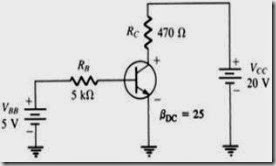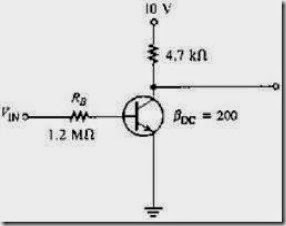# MCQs in Bipolar Junction Transistors

(Last Updated On: December 8, 2017)

This is the Multiple Choice Questions in Bipolar Junction Transistors from the book Electronic Devices – Electron Flow Version and Conventional Current Version 8th Edition by Thomas L. Floyd. If you are looking for a reviewer in Electronics Engineering this will definitely help. I can assure you that this will be a great help in reviewing the book in preparation for your Board Exam. Make sure to familiarize each and every questions to increase the chance of passing the ECE Board Exam.

### Online Questions and Answers Topic Outline

• MCQs in Bipolar Junction Transistor (BJT) Structure
• MCQs in Basic BJT Operation
• MCQs in BJT Characteristics and Parameters
• MCQs in The BJT as an Amplifier
• MCQs in The Phototransistor
• MCQs in The BJT as a Switch
• MCQs in Transistor Categories and Packaging
• MCQs in GreenTech Application 4: Solar Power

### Practice Exam Test Questions

Choose the letter of the best answer in each questions.

1. The dc load line on a family of collector characteristic curves of a transistor shows the
• A) saturation region.
• B) cutoff region.
• C) active region.
• D) all of the above

2. A transistor data sheet usually identifies βDC as

• A) hre.
• B) hfe.
• C) IC.
• D) VCE.
3. When a transistor is used as a switch, it is stable in which two distinct regions?
• A) saturation and active
• B) active and cutoff
• C) saturation and cutoff
• D) none of the above
4. For a silicon transistor, when a base-emitter junction is forward-biased, it has a nominal voltage drop of
• A) 0.7 V.
• B) 0.3 V.
• C) 0.2 V.
• D) VCC.

5. The value of βDC

• A) is fixed for any particular transistor.
• B) varies with temperature.
• C) varies with IC.
• D) varies with temperature and IC.

6. The term BJT is short for

• A) base junction transistor.
• B) binary junction transistor.
• C) both junction transistor.
• D) bipolar junction transistor.

7. A BJT has an IB of 50 µA and a βDC of 75; IC is:

• A) 375 mA
• B) 37.5 mA
• C) 3.75 mA
• D) 0.375 mA

8. A certain transistor has IC = 15 mA and IB = 167 µA; βDC is:

• A) 15
• B) 167
• C) 0.011
• D) 90
9. For normal operation of a pnp BJT, the base must be _____ with respect to the emitter and _____ with respect to the collector.
• A) positive, negative
• B) positive, positive
• C) negative, positive
• D) negative, negative
10. A transistor amplifier has a voltage gain of 100. If the input voltage is 75 mV, the output voltage is:
• A) 1.33 V
• B) 7.5 V
• C) 13.3 V
• D) 15 V
11. A 35 mV signal is applied to the base of a properly biased transistor with an r’e = 8 Ω and RC = 1 kΩ. The output signal voltage at the collector is:
• A) 3.5 V
• B) 28.57 V
• C) 4.375 V
• D) 4.375 mV

12. Refer to Figure 4-1; the value of VBE is:Figure 4-1

• A) 0.6 V
• B) 0.7 V
• C) 1.2 V
• D) 0.079 V

13. Refer to Figure 4-1; the value of VCE is:

• A) 9.9 V
• B) 9.2 V
• C) 0.7 V
• D) 19.3 V

14. Refer to Figure 4-1; the value of VBC is:

• A) 9.2 V
• B) 9.9 V
• C) –9.9 V
• D) –9.2 V

15. Refer to Figure 4-2; if VCE = 0.2 V, IC(sat) is:Figure 4-2

• A) 0.05 mA
• B) 2.085 mA
• C) 1.065 mA
• D) 7.4 mA
16. Refer to Figure 4-2. Determine the minimum value of IB that will produce saturation.
• A) 0.25 mA
• B) 5.325 A
• C) 1.065 A
• D) 10.425 A
17. Refer to Figure 4-2. Determine the minimum value of VIN from the following that will saturate this transistor.
• A) 13.21 V
• B) 12.51 V
• C) 0.7 V
• D) 9.4 V
18. Refer to Figure 4-3. The value of βDC = 100 and VIN = 8 V. Determine IC(sat).
• A) 18 mA
• B) 7.92 mA
• C) 1.8 mA
• D) 8 A
19. Refer to Figure 4-3. In this circuit βDC = 100 and VIN = 8 V. The value of RB that will produce saturation is:
• A) 92 kΩ
• B) 9.1 MΩ
• C) 100 kΩ
• D) 150 kΩ
20. Refer to Figure 4-3. The measured voltage, VCE, is 20 V. The transistor is in
• A) saturation.
• B) cutoff.
• C) normal.
• D) not enough data.
21. Refer to Figure 4-3. You measure VCE and find it nearly equal to zero. You now know that the transistor is
• A) operating in cutoff.
• B) operating normally.
• C) operating in saturation.
• D) operating below cutoff.
22. What is the order of doping, from heavily to lightly doped, for each region?
• A) base, collector, emitter
• B) emitter, collector, base
• C) emitter, base, collector
• D) collector, emitter, base

23. What are the two types of bipolar junction transistors?

• A) npn and pnp
• B) pnn and nnp
• C) ppn and nnp
• D) pts and stp

24. Which of the following is true for an npn or pnp transistor?

• A) IE = IB + IC
• B) IB = IC+ IE
• C) IC = IB + IE
• D) none of the above

25. What is the ratio of IC to IB?

• A) βDC
• B) hFE
• C) αDC
• D) either βDC or hFE, but not αDC

26. What is the ratio of IC to IE?

• A) βDC
• B) βDC / (βDC + 1)
• C) αDC
• D) either βDC / (βDC + 1) or αDC, but not βDC
27. In what range of voltages is the transistor in the linear region of its operation?
• A) 0 < VCE
• B) 0.7 < VCE < VCE(max)
• C) VCE(max) > VCE
• D) none of the above

28. What does DC vary with?

• A) IC
• B) ºC
• C) both IC and ºC
• D) IC’, but not ºC

29. What is (are) common fault(s) in a BJT-based circuit?

• A) opens or shorts internal to the transistor
• B) open bias resistor(s)
• C) external opens and shorts on the circuit board
• D) all of the above
30. What is (are) general-purpose/small-signal transistors case type(s)?
• A) TO-18
• B) TO-92
• C) TO-39
• D) TO-52
• E) all of the above
31. The magnitude of dark current in a phototransistor usually falls in what range?
• A) mA
• B) μA
• C) nA
• D) pA